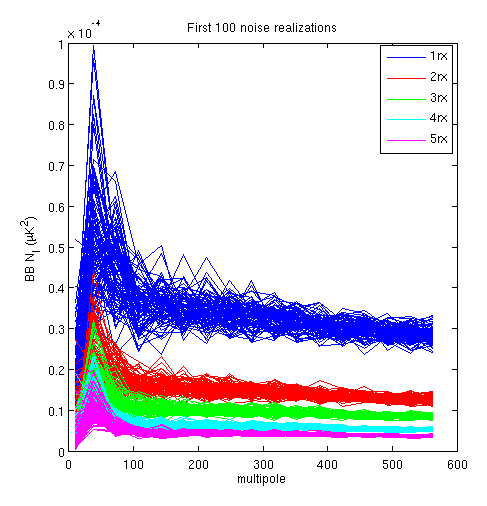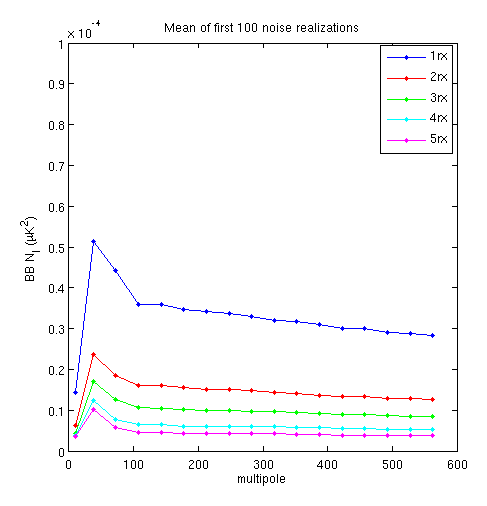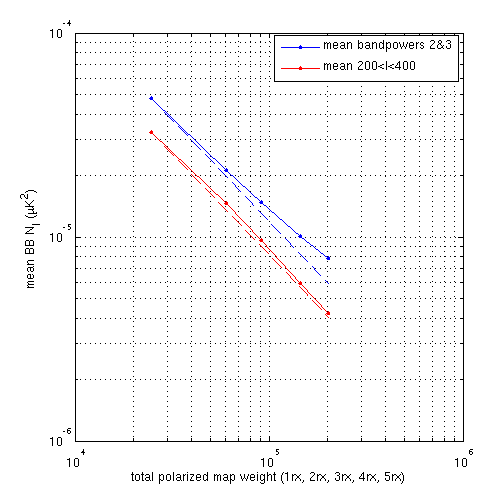## How $$N_\ell$$ integrates down with $$N_{\mathrm{det}}$$ in K2013 data

### 2020 Nov 16 - Clem Pryke

We have looked at noise integrating down with time in posts like 20180618_mapnoise. As far as I know we have not looked much at noise integrating down with number of detectors. In 2013 Keck ran with five 150GHz receivers. We store the coadded maps on a per receiver basis (jack01) offering the opportunity to accumulate to 1-receiver, 2-receiver, 3-receiver etc. maps with minimal effort.

Below are the polarization weight maps $$1/Q_{\mathrm{var}} + 1/U_{\mathrm{var}}$$ for each of the five cases. We see that rx4 is the most valuable player.

Fig1:Since these are noise realizations they contain roughly equal E and B so no special techniques are need to take angular power spectra. The left plot shows the first 100 realizations and the right plot shows the means. We see a rise to low $$\ell$$ as expected followed by a falloff in the lowest (junk) bin due to the timestream filtering. Note that the $$\ell(\ell+1)/2\pi$$ factor has been divided out to plot "raw $$N_\ell$$".

Fig2:Taking the mean of bandpowers 2&3 and the region $$200<\ell<400$$ and plotting log-log style we get the below. The dashed lines just scale the first point by the ratio of the total weights. We see that for the higher $$\ell$$ things follow the prediction closely but for lower $$\ell$$ there is some excess. The final point is about 30% higher than the extrapolation. This is presumably due to the effect of correlated noise amongst the pairs - see 20180205_cov_noise_penalty for a related posting.

Fig3:An interesting next step would be to do the same thing with BICEP3. A special per tile coadd could be built and then the tiles added up in an order that builds to full sky coverage quickly and then maintains roughly uniform sky coverage.

### Code

% look at N_ell as a function of N_det

% Keck 2012/13 was 5x150GHz rx

% look at ind rx maps
map=make_map(ac,m);
plot_map(m,map(2).Pwitime); colorbar

% build cumulative maps and record total weight
for i=1:5
map(i)=make_map(acc,m);
% Pitime field has problems due to use of dwmax field - a few crazy high weights messes it up -
% now there shouldn't be any crazy high weights but...
it(i)=nansum(map(i).Pwitime(:));
% for total weight it might make more sense to use 1/Qvar+1/Uvar
tw(i)=nansum(1./map(i).Qvar(:)+1./map(i).Uvar(:));
end

setwinsize(gcf,800,500); clf
subplot(3,2,1); plot_map(m,1./map(1).Qvar+1./map(1).Uvar); colorbar; title('1rx');
subplot(3,2,2); plot_map(m,1./map(2).Qvar+1./map(2).Uvar); colorbar; title('2rx');
subplot(3,2,3); plot_map(m,1./map(3).Qvar+1./map(3).Uvar); colorbar; title('3rx');
subplot(3,2,4); plot_map(m,1./map(4).Qvar+1./map(4).Uvar); colorbar; title('4rx');
subplot(3,2,5); plot_map(m,1./map(5).Qvar+1./map(5).Uvar); colorbar; title('5rx');
mkgif('fig1.gif',1);

return

% build 1,2,3,4,5 rx N_ell
uk=get_ukpervolt('201308','keck');
for i=1:5
apsopt.ukpervolt=NaN*ones(1,5);
apsopt.ukpervolt(1:i)=uk;
apsopt.daughter=sprintf('%1drx',i);
reduc_makeaps('maps/1351/0??6_b_filtp3_weight3_gs_dp1100_jack01.mat',apsopt);
end

reduc_makesimset('1351/0??6_b_filtp3_weight3_gs_dp1100_jack01_overrx_1rx.mat');
reduc_makesimset('1351/0??6_b_filtp3_weight3_gs_dp1100_jack01_overrx_2rx.mat');
reduc_makesimset('1351/0??6_b_filtp3_weight3_gs_dp1100_jack01_overrx_3rx.mat');
reduc_makesimset('1351/0??6_b_filtp3_weight3_gs_dp1100_jack01_overrx_4rx.mat');
reduc_makesimset('1351/0??6_b_filtp3_weight3_gs_dp1100_jack01_overrx_5rx.mat');

return

sf=2*pi./(aps.l.^2);

setwinsize(gcf,500,500); clf
s=4;
h1=plot(aps.l,squeeze(repmat(sf,[1,1,size(aps1.Cs_l,3)]).*aps1.Cs_l(:,s,:)),'b');
hold on
h2=plot(aps.l,squeeze(repmat(sf,[1,1,size(aps1.Cs_l,3)]).*aps2.Cs_l(:,s,:)),'r');
h3=plot(aps.l,squeeze(repmat(sf,[1,1,size(aps1.Cs_l,3)]).*aps3.Cs_l(:,s,:)),'g');
h4=plot(aps.l,squeeze(repmat(sf,[1,1,size(aps1.Cs_l,3)]).*aps4.Cs_l(:,s,:)),'c');
h5=plot(aps.l,squeeze(repmat(sf,[1,1,size(aps1.Cs_l,3)]).*aps5.Cs_l(:,s,:)),'m');
hold off
xlabel('multipole'); ylabel('BB N_l (\muK^2)');
legend([h1(1),h2(1),h3(1),h4(1),h5(1)],{'1rx','2rx','3rx','4rx','5rx'});
title('First 100 noise realizations');
mkgif('fig2a.gif',1);

aps1.Cs_l=mean(aps1.Cs_l,3);
aps2.Cs_l=mean(aps2.Cs_l,3);
aps3.Cs_l=mean(aps3.Cs_l,3);
aps4.Cs_l=mean(aps4.Cs_l,3);
aps5.Cs_l=mean(aps5.Cs_l,3);

setwinsize(gcf,500,500); clf
h1=plot(aps.l,sf.*aps1.Cs_l(:,s),'b.-');
hold on
h2=plot(aps.l,sf.*aps2.Cs_l(:,s),'r.-');
h3=plot(aps.l,sf.*aps3.Cs_l(:,s),'g.-');
h4=plot(aps.l,sf.*aps4.Cs_l(:,s),'c.-');
h5=plot(aps.l,sf.*aps5.Cs_l(:,s),'m.-');
hold off
ylim([0,1e-4]);
xlabel('multipole'); ylabel('BB N_l (\muK^2)');
legend([h1(1),h2(1),h3(1),h4(1),h5(1)],{'1rx','2rx','3rx','4rx','5rx'});
title('Mean of first 100 noise realizations');
mkgif('fig2b.gif',1);

i1=aps.l>10&aps.l<100;
i2=aps.l>200&aps.l<400;

of(1)=mean(sf(i1).*aps1.Cs_l(i1,s));
of(2)=mean(sf(i1).*aps2.Cs_l(i1,s));
of(3)=mean(sf(i1).*aps3.Cs_l(i1,s));
of(4)=mean(sf(i1).*aps4.Cs_l(i1,s));
of(5)=mean(sf(i1).*aps5.Cs_l(i1,s));

wn(1)=mean(sf(i2).*aps1.Cs_l(i2,s));
wn(2)=mean(sf(i2).*aps2.Cs_l(i2,s));
wn(3)=mean(sf(i2).*aps3.Cs_l(i2,s));
wn(4)=mean(sf(i2).*aps4.Cs_l(i2,s));
wn(5)=mean(sf(i2).*aps5.Cs_l(i2,s));

setwinsize(gcf,500,500); clf
loglog(tw,of,'b.-');
hold on
loglog(tw,wn,'r.-');
% extrapolate forward from the first point based on relative map weight
ofp(1)=of(1); ofp(2:5)=of(1)*tw(1)./tw(2:5);
wnp(1)=wn(1); wnp(2:5)=wn(1)*tw(1)./tw(2:5);
plot(tw,ofp,'b--')
plot(tw,wnp,'r--')
hold off
grid
xlabel('total polarized map weight (1rx, 2rx, 3rx, 4rx, 5rx)'); ylabel('mean BB N_l (\muK^2)');
legend({'mean bandpowers 2&3','mean 200